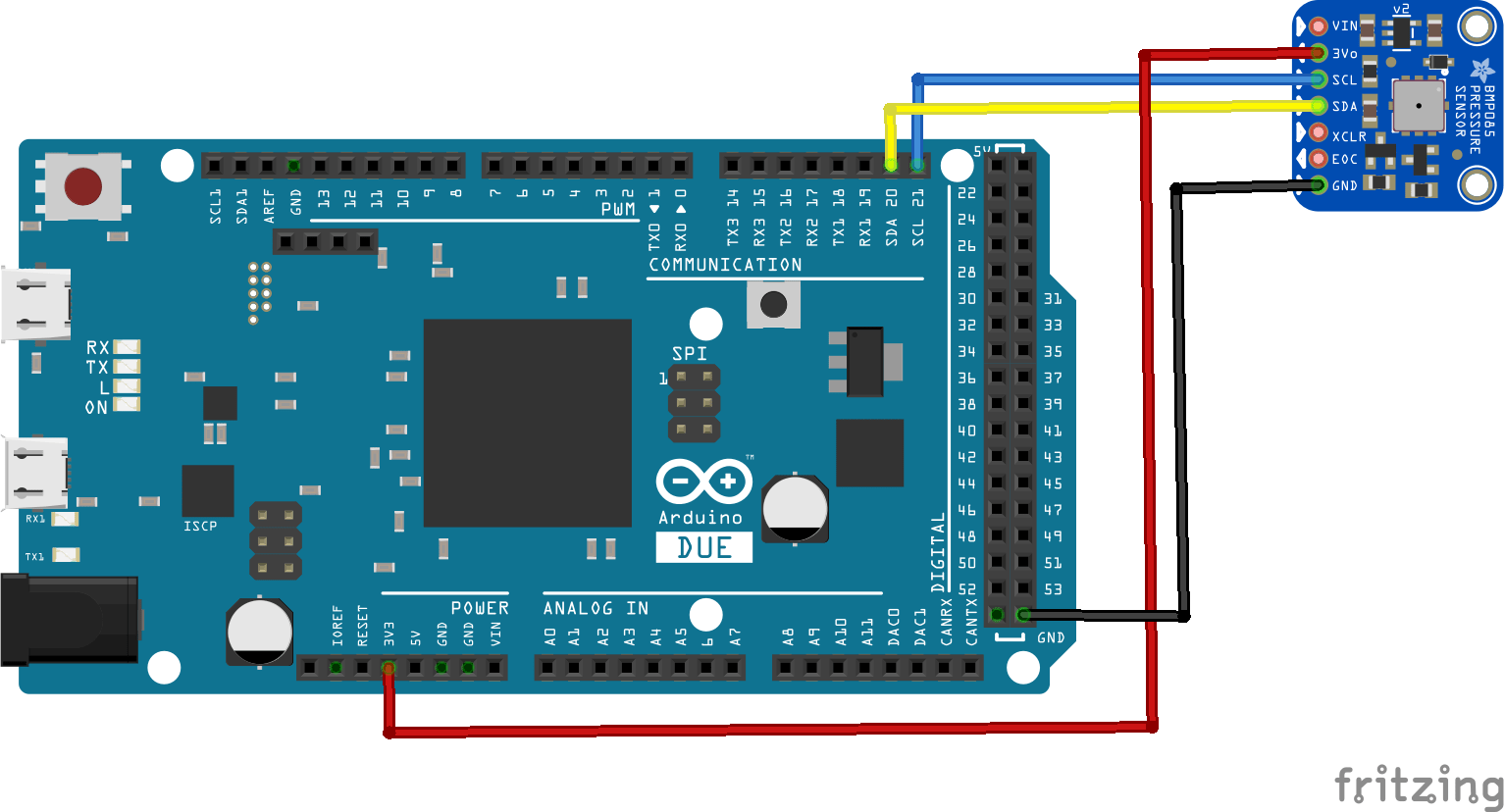# Arduino Due and BMP085 example

In this example we connect a BMp085 sensor to an Arduino Due, you can use this to measure barometric pressure and temperature

This sensor has been replaced by some newer versions but there are still many modules available so here is an example on how to use it

Schematics

Easy to connect up, you can see this in the layout below.Code

[codesyntax lang=”cpp”]

```#include <Wire.h>

void setup()
{
Serial.begin(9600);
if (!bmp.begin())
{
Serial.println("Could not find a BMP085 sensor!");
while (1) {}
}
}

void loop()
{
Serial.print("Temperature = ");
Serial.println(" *C");

Serial.print("Pressure = ");
Serial.println(" Pa");

Serial.print("Altitude = ");
Serial.println(" meters");

Serial.print("Real altitude = ");
Serial.println(" meters");

Serial.println();
delay(500);
}```

[/codesyntax]

Output

Open the serial monitor and you should see something like this

Temperature = 27.30 *C
Pressure = 99058 Pa
Altitude = 190.05 meters
Real altitude = 204.46 meters

Temperature = 27.60 *C
Pressure = 99064 Pa
Altitude = 189.63 meters
Real altitude = 204.29 meters

Temperature = 27.90 *C
Pressure = 99062 Pa
Altitude = 189.54 meters
Real altitude = 203.78 meters

This div height required for enabling the sticky sidebar